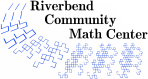# The Doorbell Rang

### Description

After reading "The Doorbell Rang" by Pat Hutchins, students explore fractional representations for a set of cookies. Students communicate their thinking through modeling, discussions, and writing.

### Levels

• Grades 3 through 6.

up to 1 hour

### Activity Time

five 30 to 60 minute lessons.

### Topics

• Interpreting fractions in context
• Fraction models
• Unit fractions
• Fraction equivalence
• Comparing fractions
• Adding fractions with like denominators
• Adding fractions with unlike denominators
• Multiplying whole numbers and fractions

### Goals

• Students will be able to interpret fractions as equal groups and as ratios.
• Students will be able to correctly determine fractions of a set.
• Students will be able to identify equivalent fractions and justify how they know those fractions are equivalent.
• Students will be able to compare fractions.
• Students will be able to add fractions with common denominators.
• Students will begin to explore addition with unlike denominators and use estimation of sums.
• Students will understand that multiplying a fraction by a whole number can be understood as repeated addition.

### Materials

• a copy of "The Doorbell Rang" by Pat Hutchins
• chart paper
• bags of cookie cereal with 12 pieces (one for each student)
• counters (24 per student)
• sheets of manila paper (1 per student)
• copies of activity handouts (1 set per student)

### Prerequisites

• Students should be able to count, read, and write.

### Authors

Cheryl A. Nix, M.S. and Amanda Katharine Serenevy, Ph.D.

### National Common Core Standards

 3.NF.1 Understand a fraction 1/b as the quantity formed by one part when a whole is partitioned into b equal parts; understand a/b as a pieces of size 1/b. 3.NF.3 Explain equivalence of fractions in special cases and compare fractions by reasoning about their size. 4.NF.1 Explain why a fraction a/b is equivalent to a fraction (nxa)/(nxb) by using visual fraction models. 4.NF.2 Compare two fractions with different numerators and different denominators by creating common denominators or numerators or by comparing to benchmark fractions. 4.NF.3 Understand a fraction a/b with a>1 as the sum of fractions 1/b, and add and subtract mixed numbers with like denominators. 4.NF.4 Apply and extend previous understandings of multiplication to multiply a fraction by a whole number (including results that are improper fractions). 5.NF.1 Add and subtract fractions with unlike denominators (including mixed numbers) by replacing given fractions with equivalent fractions in such a way as to produce an equivalent sum or difference of fractions with like denominators. 5.NF.2 Solve word problems involving addition and subtraction of fractions referring to the same whole, including cases of unlike denominators, e.g. by using visual fraction models or equations to represent the problem. Use benchmark fractions to estimate mentally and assess reasonableness of answers.
Riverbend Community Math Center
hello@riverbendmath.org
http://riverbendmath.org
(574) 339-9111This work placed into the public domain by the Riverbend Community Math Center.